Скачать презентацию Statistics for Business and Economics Chapter 9 Categorical

8def9eca15db9d5a98aaa98cb851d7d4.ppt

• Количество слайдов: 56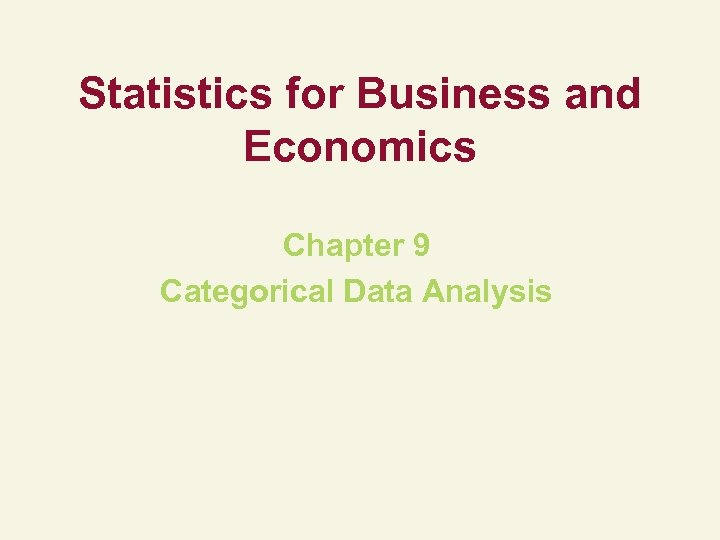Statistics for Business and Economics Chapter 9 Categorical Data Analysis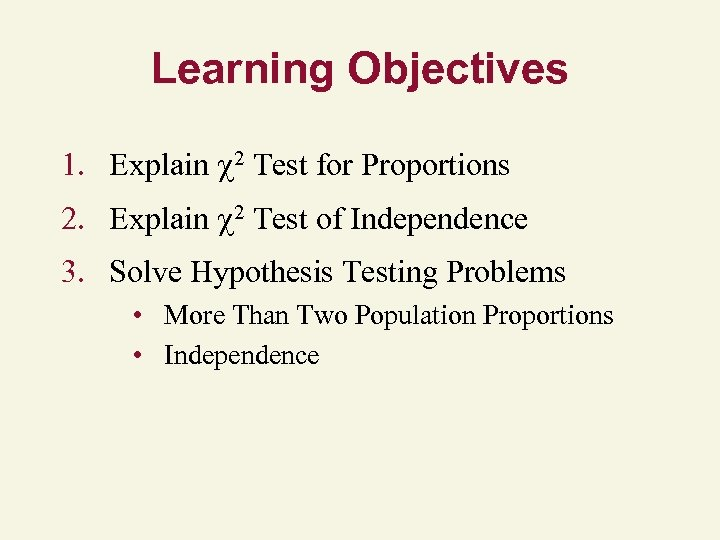Learning Objectives 1. Explain 2 Test for Proportions 2. Explain 2 Test of Independence 3. Solve Hypothesis Testing Problems • More Than Two Population Proportions • Independence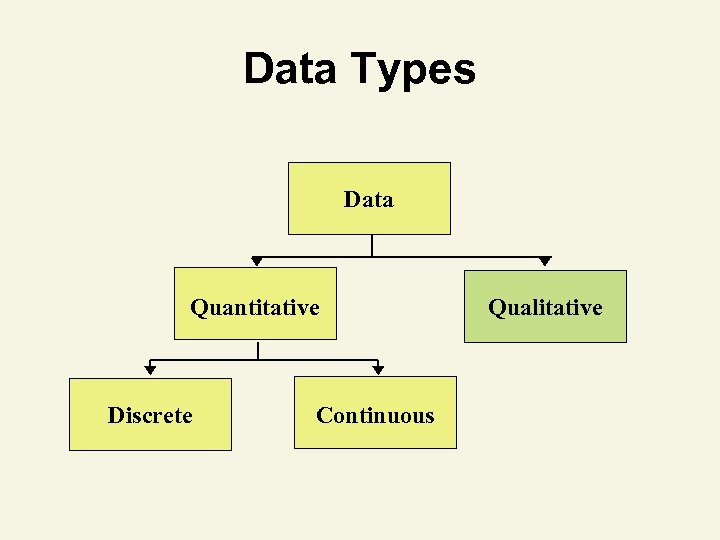Data Types Data Quantitative Discrete Continuous Qualitative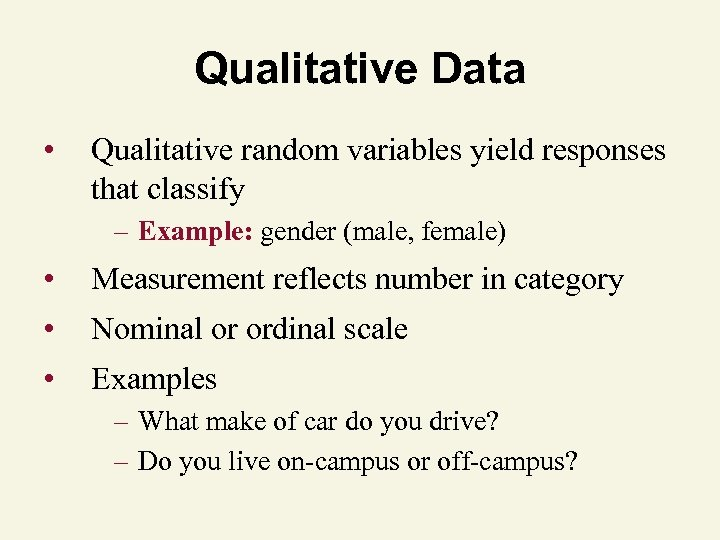Qualitative Data • Qualitative random variables yield responses that classify – Example: gender (male, female) • Measurement reflects number in category • Nominal or ordinal scale • Examples – What make of car do you drive? – Do you live on-campus or off-campus?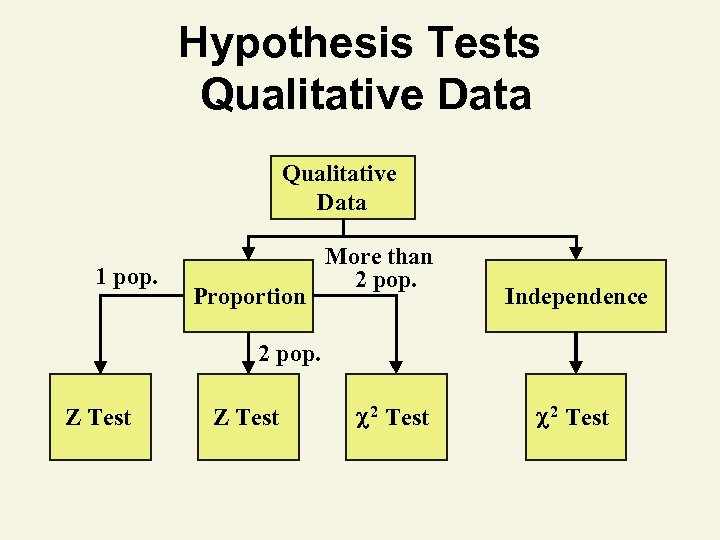Hypothesis Tests Qualitative Data 1 pop. Proportion More than 2 pop. Independence 2 pop. Z Test 2 Test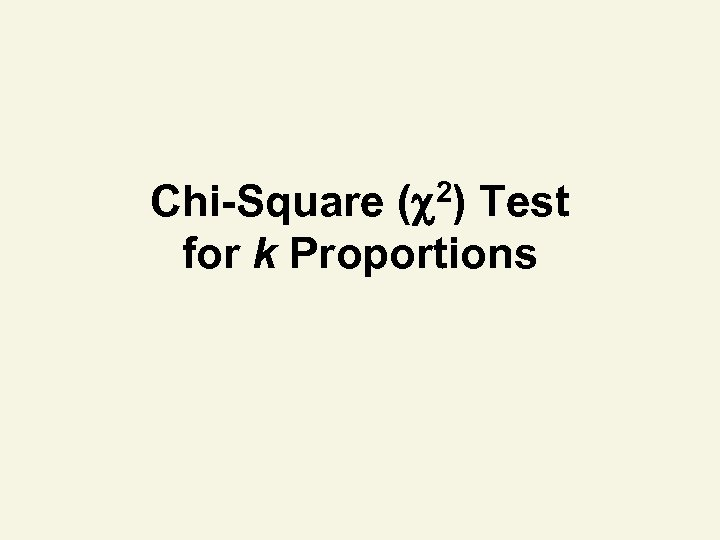Chi-Square ( 2) Test for k Proportions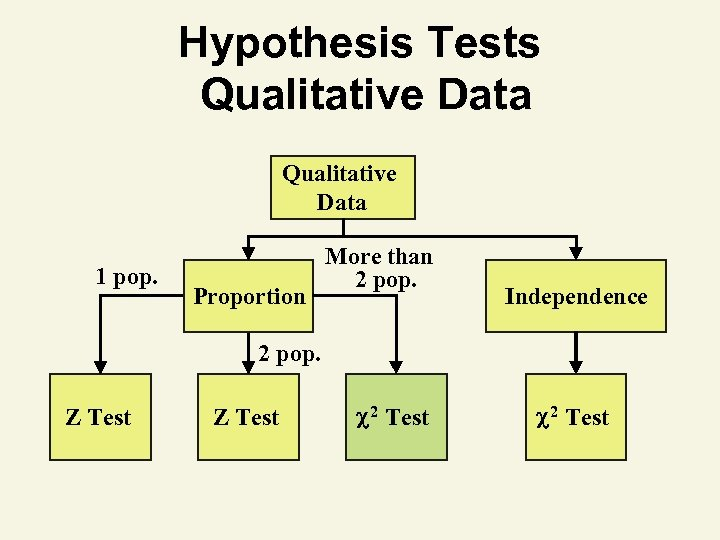Hypothesis Tests Qualitative Data 1 pop. Proportion More than 2 pop. Independence 2 pop. Z Test 2 Test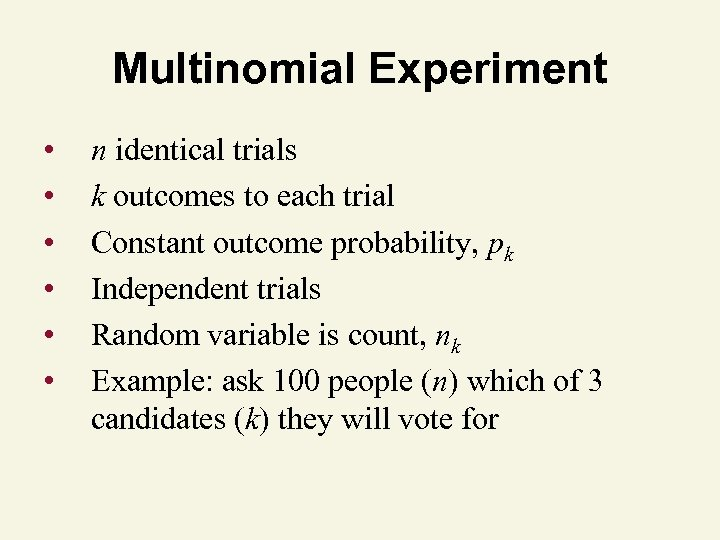Multinomial Experiment • • • n identical trials k outcomes to each trial Constant outcome probability, pk Independent trials Random variable is count, nk Example: ask 100 people (n) which of 3 candidates (k) they will vote for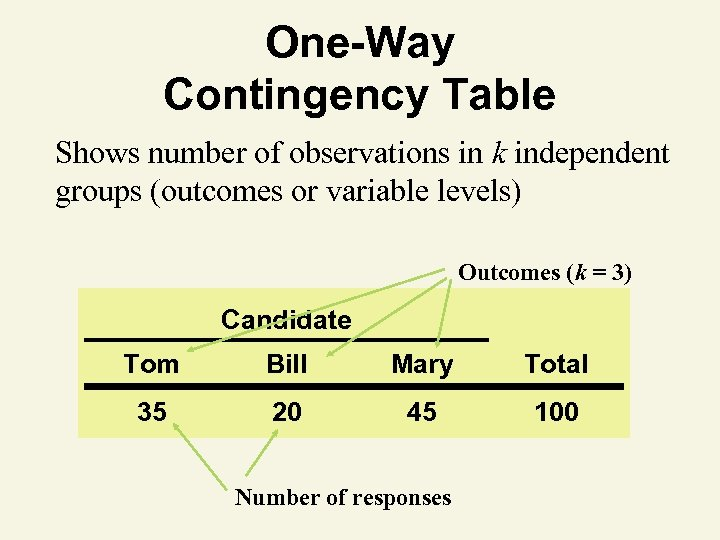One-Way Contingency Table Shows number of observations in k independent groups (outcomes or variable levels) Outcomes (k = 3) Candidate Tom Bill Mary Total 35 20 45 100 Number of responses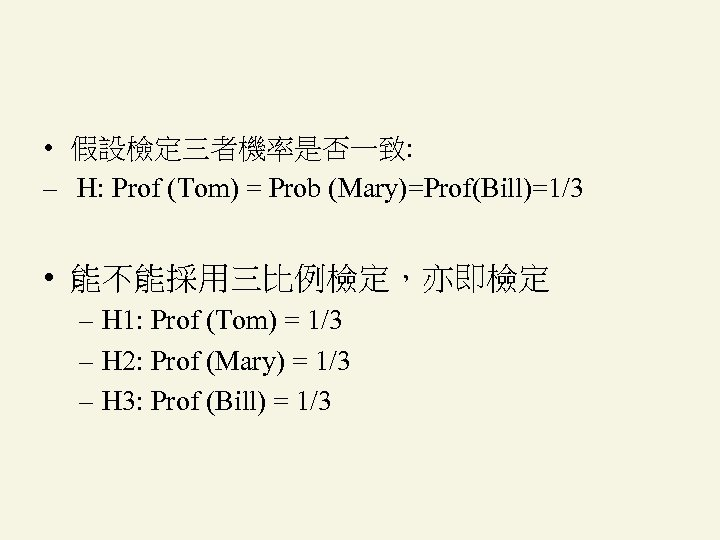• 假設檢定三者機率是否一致: – H: Prof (Tom) = Prob (Mary)=Prof(Bill)=1/3 • 能不能採用三比例檢定，亦即檢定 – H 1: Prof (Tom) = 1/3 – H 2: Prof (Mary) = 1/3 – H 3: Prof (Bill) = 1/3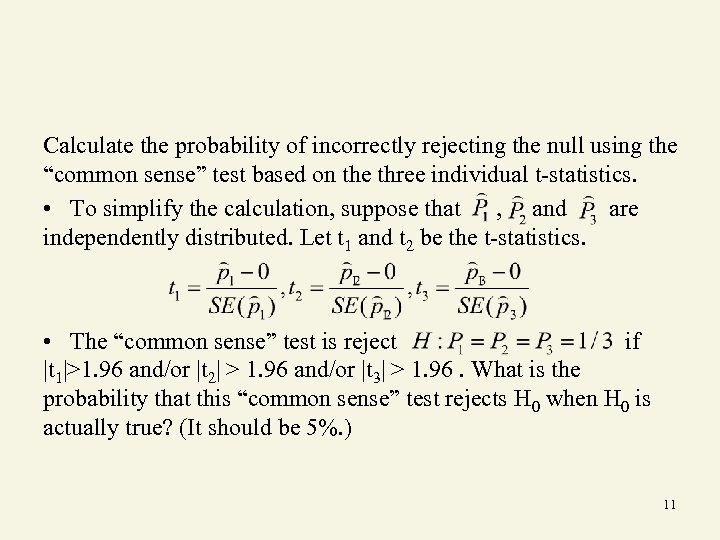Calculate the probability of incorrectly rejecting the null using the “common sense” test based on the three individual t-statistics. • To simplify the calculation, suppose that , and are independently distributed. Let t 1 and t 2 be the t-statistics. • The “common sense” test is reject if |t 1|>1. 96 and/or |t 2| > 1. 96 and/or |t 3| > 1. 96. What is the probability that this “common sense” test rejects H 0 when H 0 is actually true? (It should be 5%. ) 11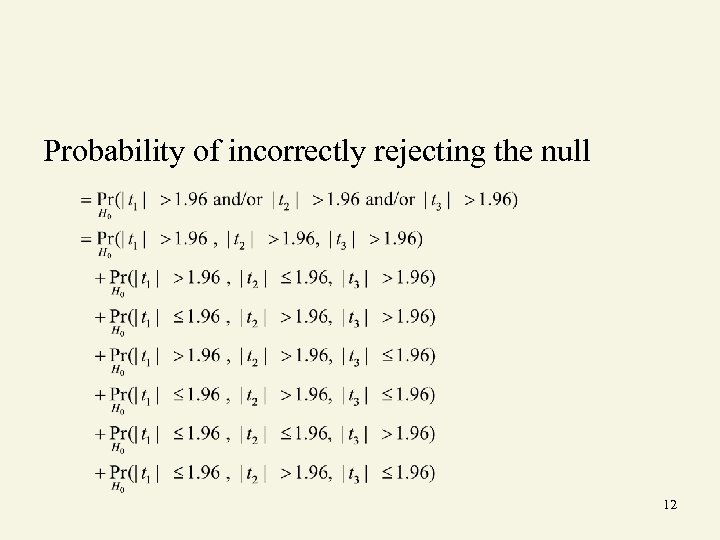Probability of incorrectly rejecting the null 12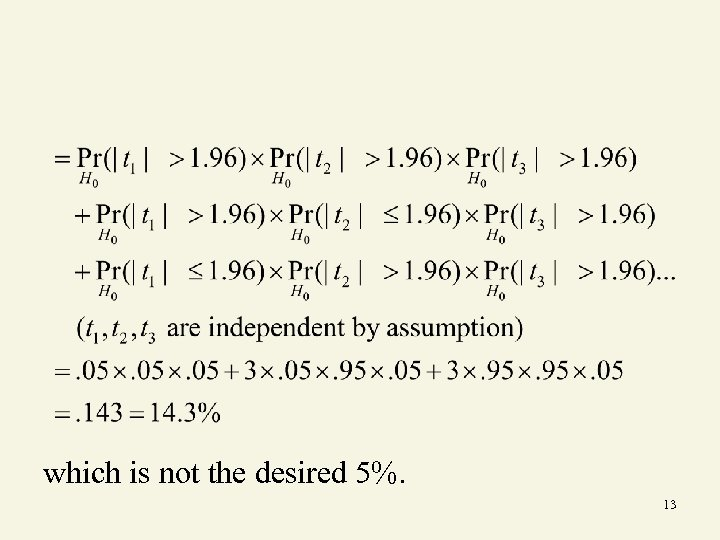which is not the desired 5%. 13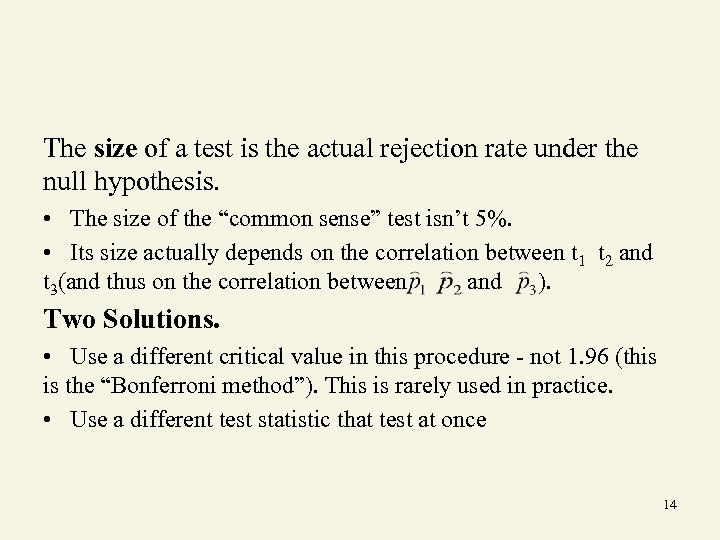The size of a test is the actual rejection rate under the null hypothesis. • The size of the “common sense” test isn’t 5%. • Its size actually depends on the correlation between t 1 t 2 and t 3(and thus on the correlation between and ). Two Solutions. • Use a different critical value in this procedure - not 1. 96 (this is the “Bonferroni method”). This is rarely used in practice. • Use a different test statistic that test at once 14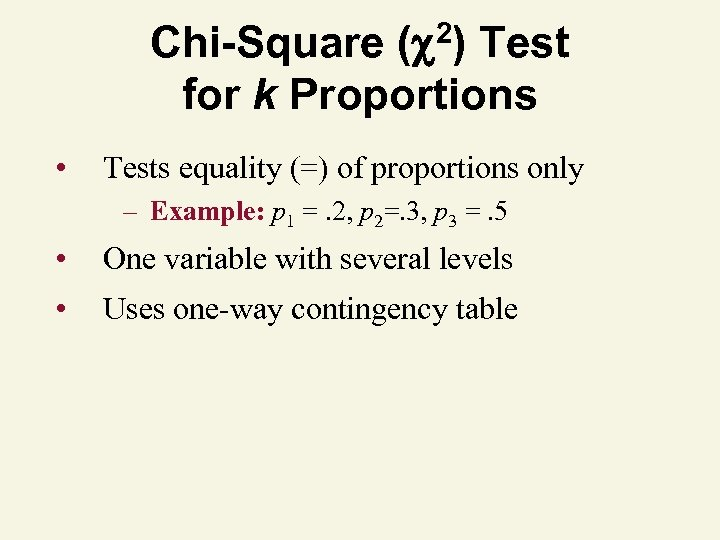2) ( Chi-Square Test for k Proportions • Tests equality (=) of proportions only – Example: p 1 =. 2, p 2=. 3, p 3 =. 5 • One variable with several levels • Uses one-way contingency table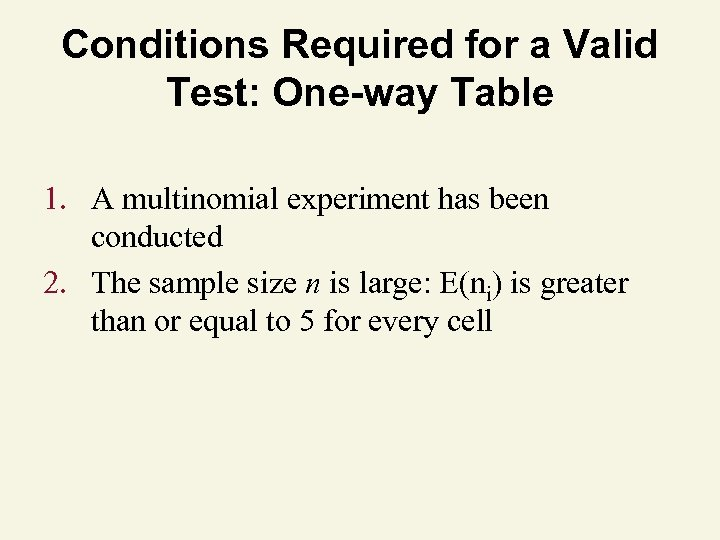Conditions Required for a Valid Test: One-way Table 1. A multinomial experiment has been conducted 2. The sample size n is large: E(ni) is greater than or equal to 5 for every cell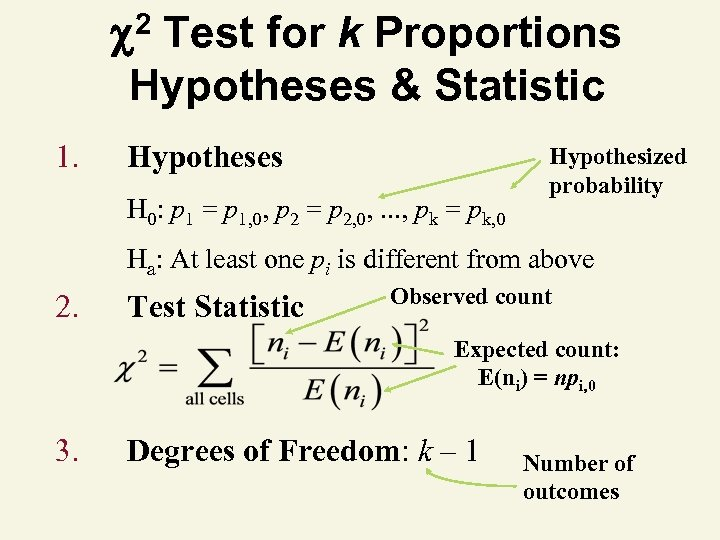2 Test for k Proportions Hypotheses & Statistic 1. Hypotheses H 0: p 1 = p 1, 0, p 2 = p 2, 0, . . . , pk = pk, 0 Hypothesized probability Ha: At least one pi is different from above 2. Test Statistic Observed count Expected count: E(ni) = npi, 0 3. Degrees of Freedom: k – 1 Number of outcomes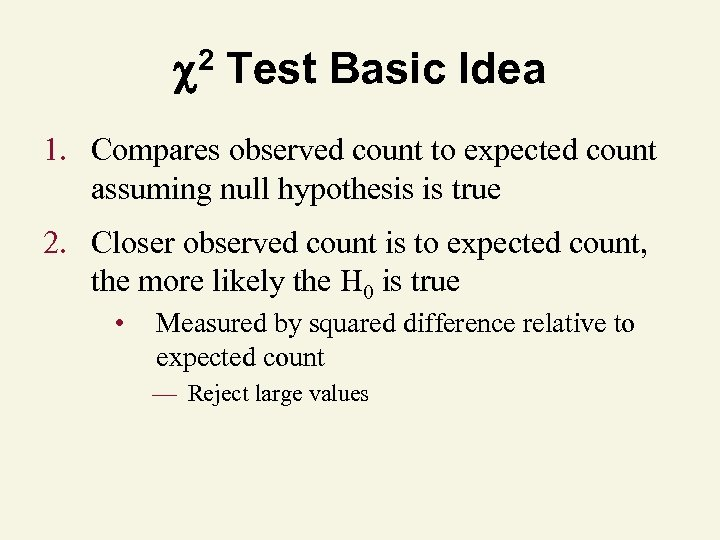2 Test Basic Idea 1. Compares observed count to expected count assuming null hypothesis is true 2. Closer observed count is to expected count, the more likely the H 0 is true • Measured by squared difference relative to expected count — Reject large values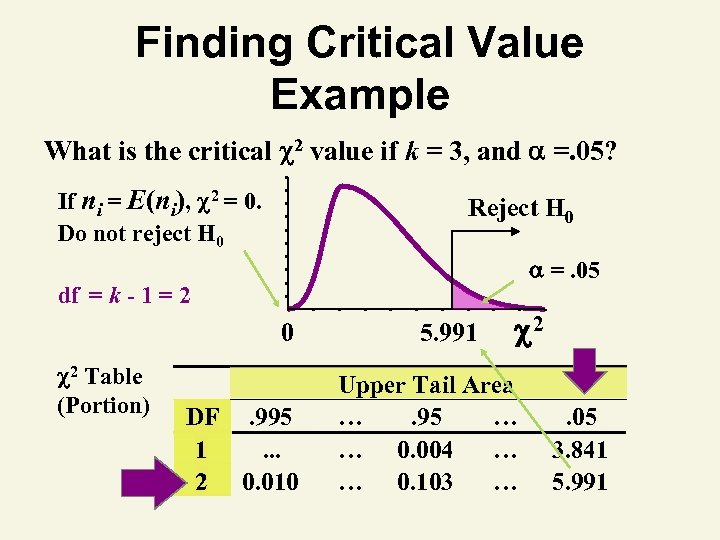Finding Critical Value Example What is the critical 2 value if k = 3, and =. 05? If ni = E(ni), 2 = 0. Do not reject H 0 Reject H 0 =. 05 df = k - 1 = 2 0 2 Table (Portion) DF. 995 1. . . 2 0. 010 5. 991 2 Upper Tail Area …. 95 … … 0. 004 … … 0. 103 … . 05 3. 841 5. 991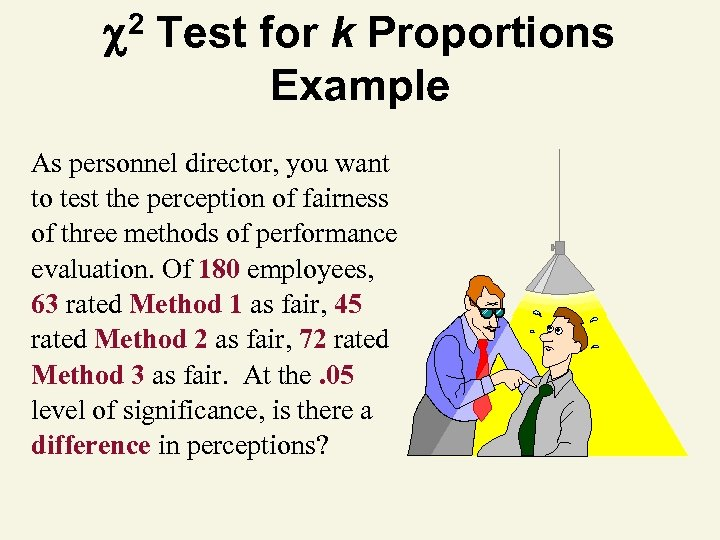2 Test for k Proportions Example As personnel director, you want to test the perception of fairness of three methods of performance evaluation. Of 180 employees, 63 rated Method 1 as fair, 45 rated Method 2 as fair, 72 rated Method 3 as fair. At the. 05 level of significance, is there a difference in perceptions?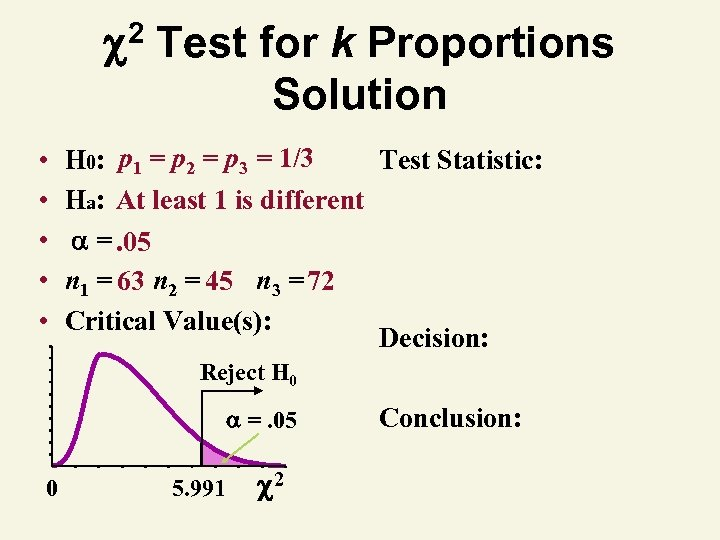2 • • • Test for k Proportions Solution H 0: p 1 = p 2 = p 3 = 1/3 Test Statistic: Ha: At least 1 is different =. 05 n 1 = 63 n 2 = 45 n 3 = 72 Critical Value(s): Decision: Reject H 0 =. 05 0 5. 991 2 Conclusion: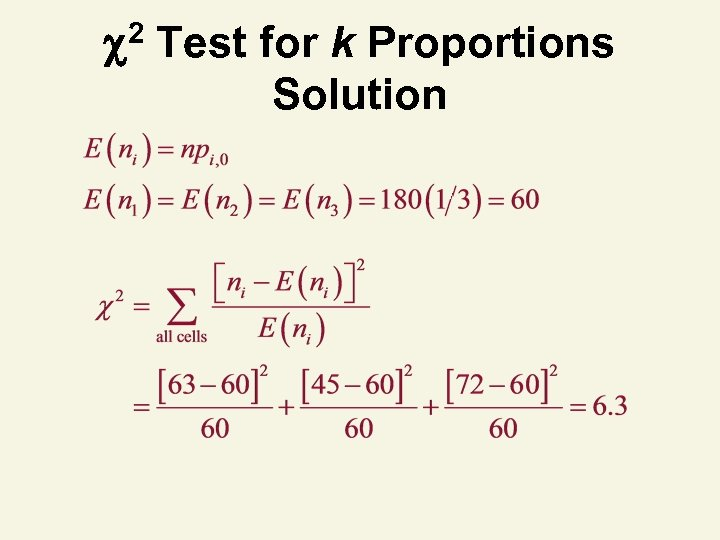2 Test for k Proportions Solution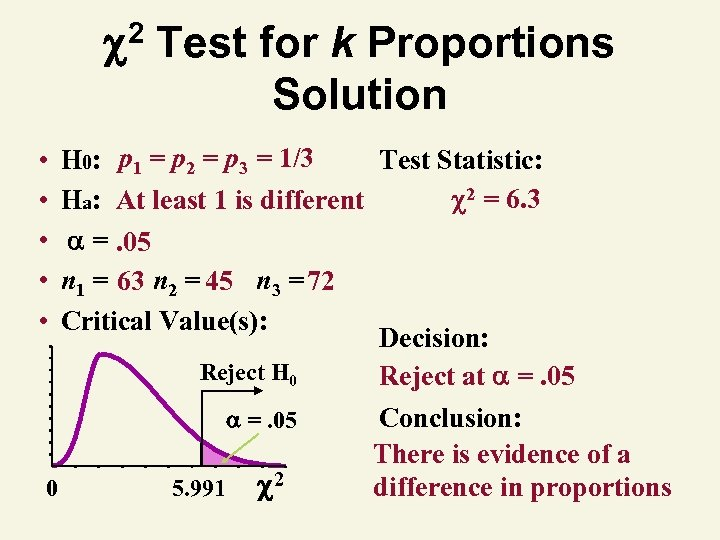2 • • • Test for k Proportions Solution H 0: p 1 = p 2 = p 3 = 1/3 Test Statistic: 2 = 6. 3 Ha: At least 1 is different =. 05 n 1 = 63 n 2 = 45 n 3 = 72 Critical Value(s): Decision: Reject H 0 Reject at =. 05 0 5. 991 2 Conclusion: There is evidence of a difference in proportions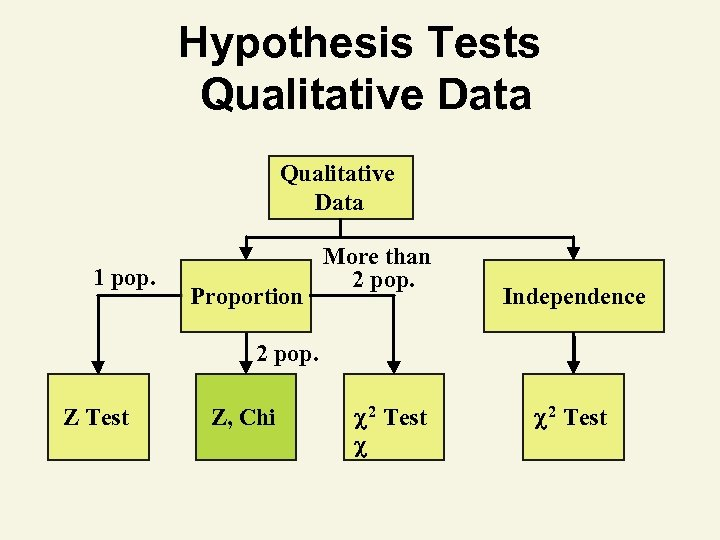Hypothesis Tests Qualitative Data 1 pop. Proportion More than 2 pop. Independence 2 pop. Z Test Z, Chi 2 Test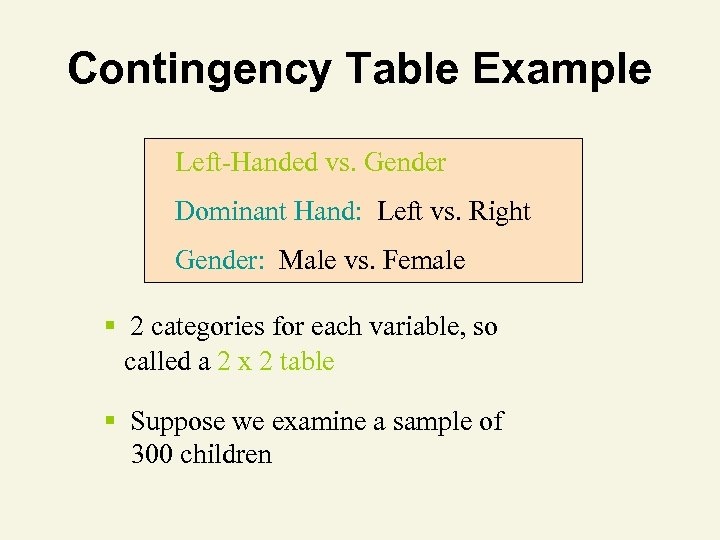Contingency Table Example Left-Handed vs. Gender Dominant Hand: Left vs. Right Gender: Male vs. Female § 2 categories for each variable, so called a 2 x 2 table § Suppose we examine a sample of 300 children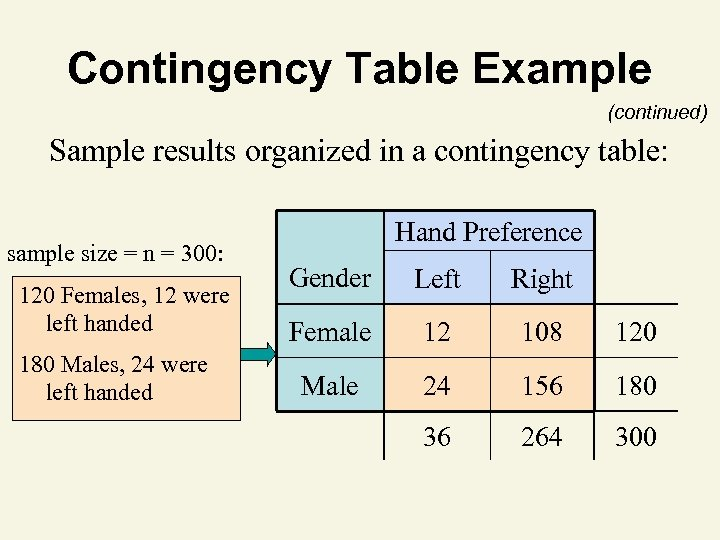Contingency Table Example (continued) Sample results organized in a contingency table: sample size = n = 300: 120 Females, 12 were left handed 180 Males, 24 were left handed Hand Preference Gender Left Right Female 12 108 120 Male 24 156 180 36 264 300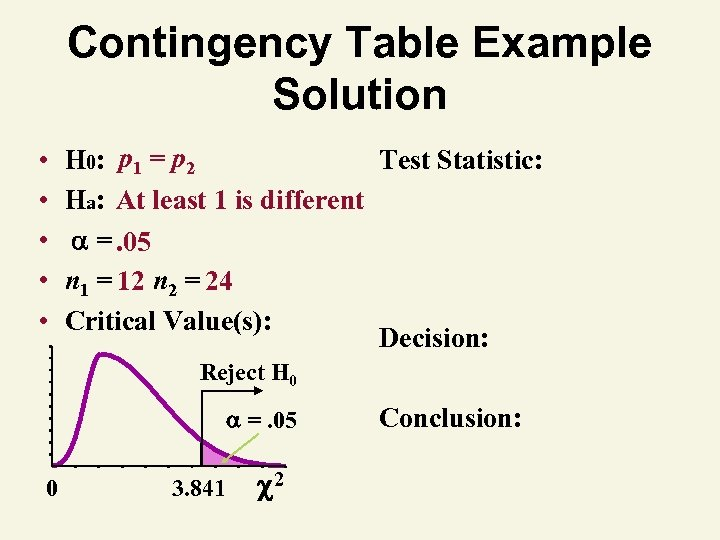Contingency Table Example Solution • • • H 0: p 1 = p 2 Test Statistic: Ha: At least 1 is different =. 05 n 1 = 12 n 2 = 24 Critical Value(s): Decision: Reject H 0 =. 05 0 3. 841 2 Conclusion: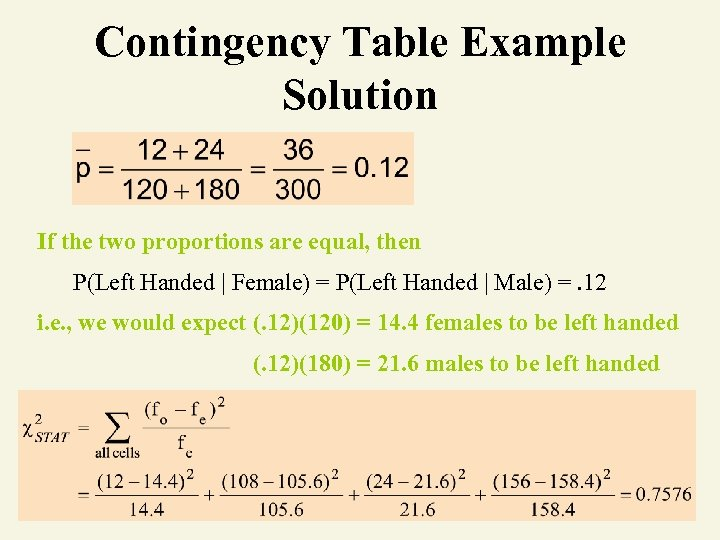Contingency Table Example Solution If the two proportions are equal, then P(Left Handed | Female) = P(Left Handed | Male) =. 12 i. e. , we would expect (. 12)(120) = 14. 4 females to be left handed (. 12)(180) = 21. 6 males to be left handed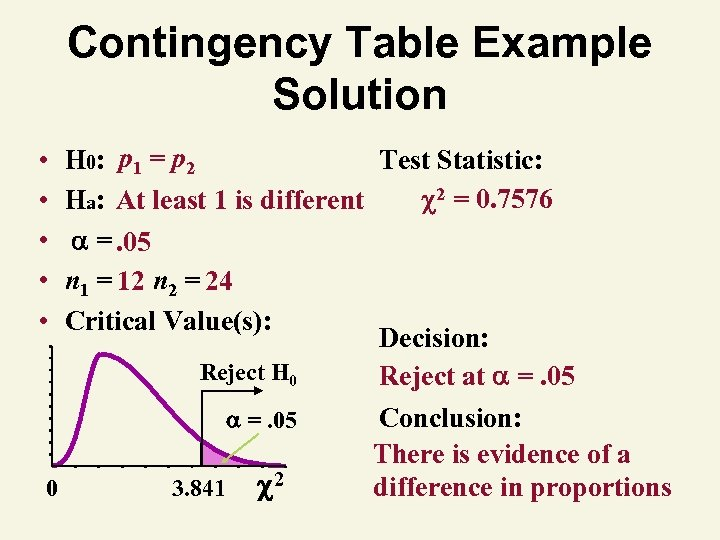Contingency Table Example Solution • • • H 0: p 1 = p 2 Test Statistic: 2 = 0. 7576 Ha: At least 1 is different =. 05 n 1 = 12 n 2 = 24 Critical Value(s): Decision: Reject H 0 Reject at =. 05 0 3. 841 2 Conclusion: There is evidence of a difference in proportions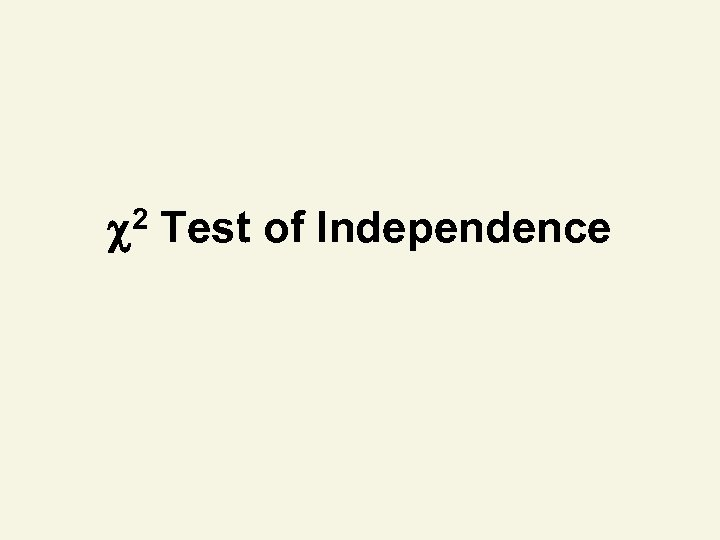2 Test of Independence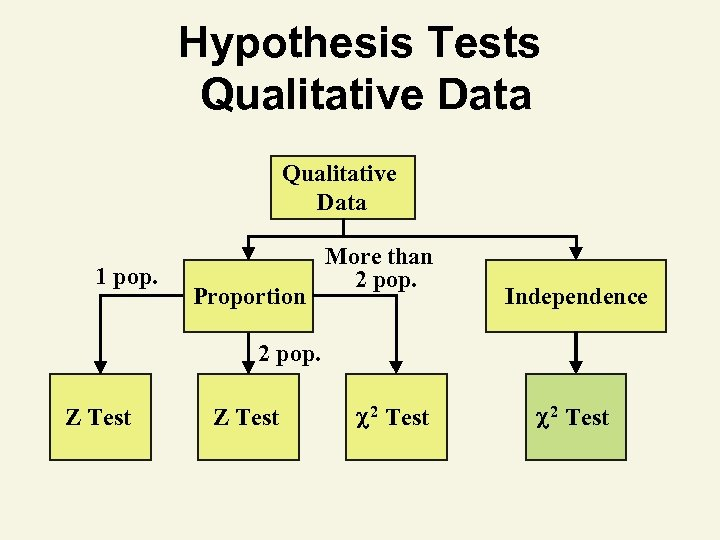Hypothesis Tests Qualitative Data 1 pop. Proportion More than 2 pop. Independence 2 pop. Z Test 2 Test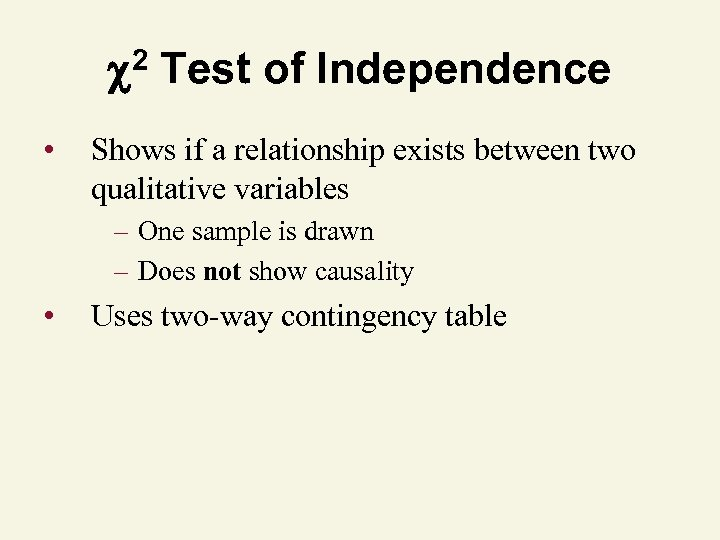2 Test of Independence • Shows if a relationship exists between two qualitative variables – One sample is drawn – Does not show causality • Uses two-way contingency table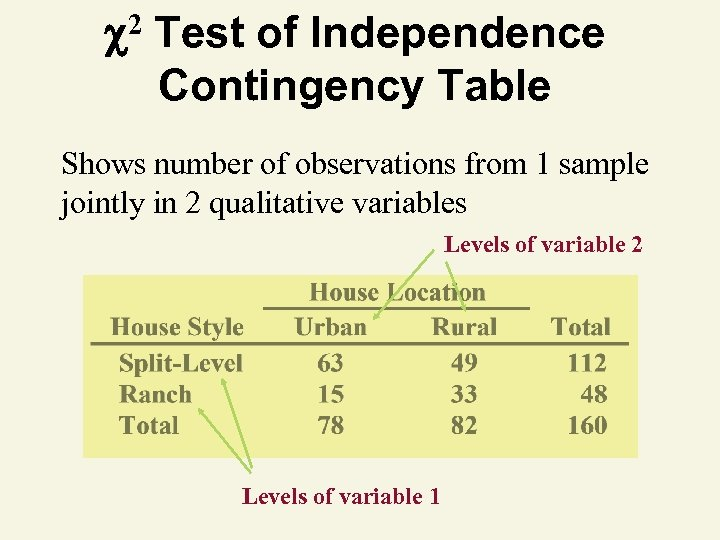2 Test of Independence Contingency Table Shows number of observations from 1 sample jointly in 2 qualitative variables Levels of variable 2 Levels of variable 1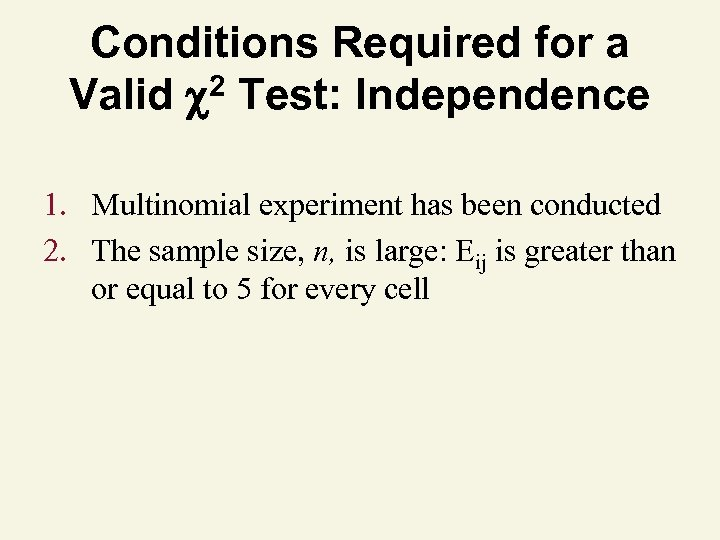Conditions Required for a 2 Test: Independence Valid 1. Multinomial experiment has been conducted 2. The sample size, n, is large: Eij is greater than or equal to 5 for every cell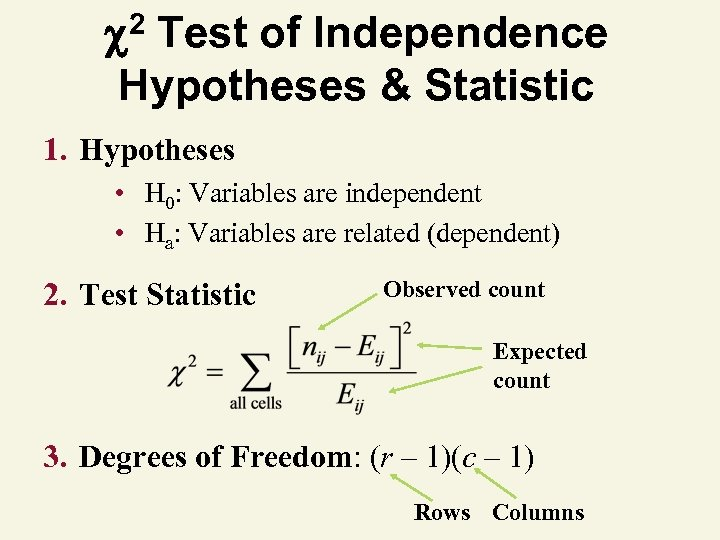2 Test of Independence Hypotheses & Statistic 1. Hypotheses • H 0: Variables are independent • Ha: Variables are related (dependent) 2. Test Statistic Observed count Expected count 3. Degrees of Freedom: (r – 1)(c – 1) Rows Columns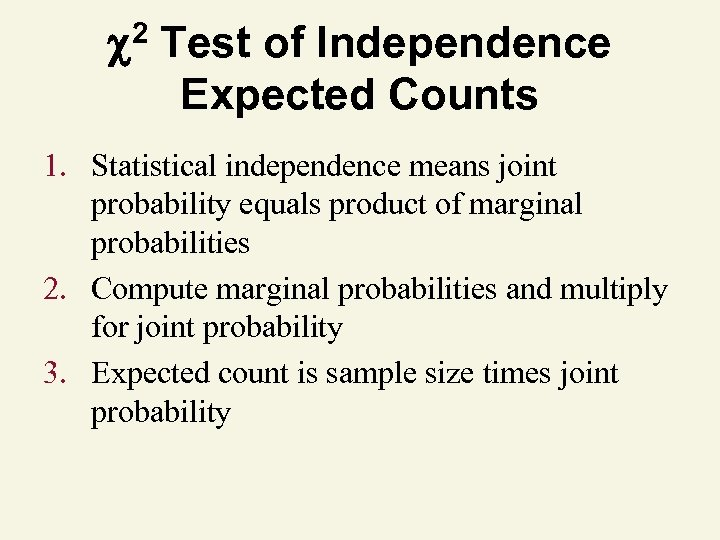2 Test of Independence Expected Counts 1. Statistical independence means joint probability equals product of marginal probabilities 2. Compute marginal probabilities and multiply for joint probability 3. Expected count is sample size times joint probability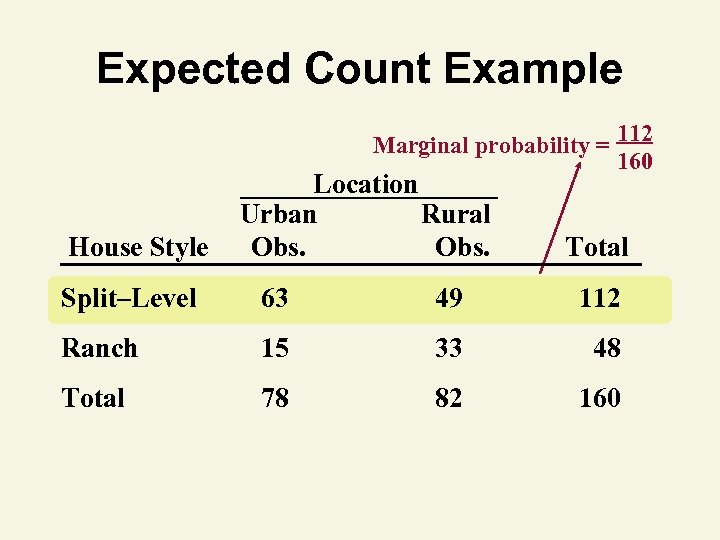Expected Count Example Marginal probability = 112 160 House Style Location Urban Rural Obs. Total Split–Level 63 49 112 Ranch 15 33 48 Total 78 82 160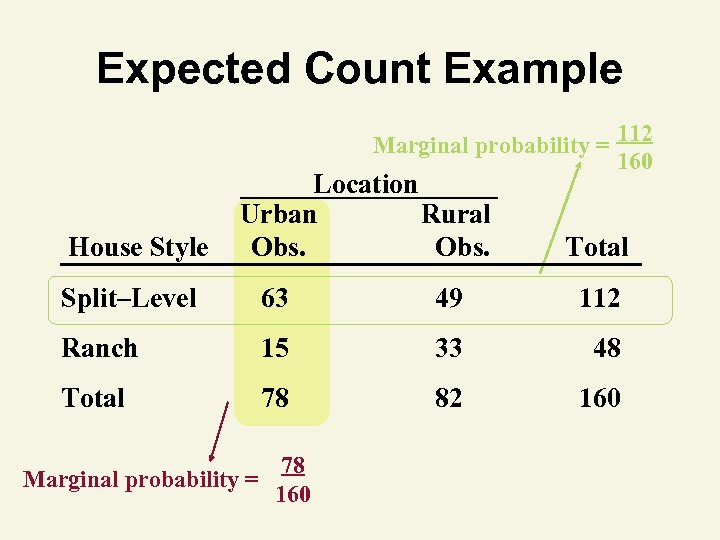Expected Count Example Marginal probability = 112 160 House Style Location Urban Rural Obs. Total Split–Level 63 49 112 Ranch 15 33 48 Total 78 82 160 Marginal probability = 78 160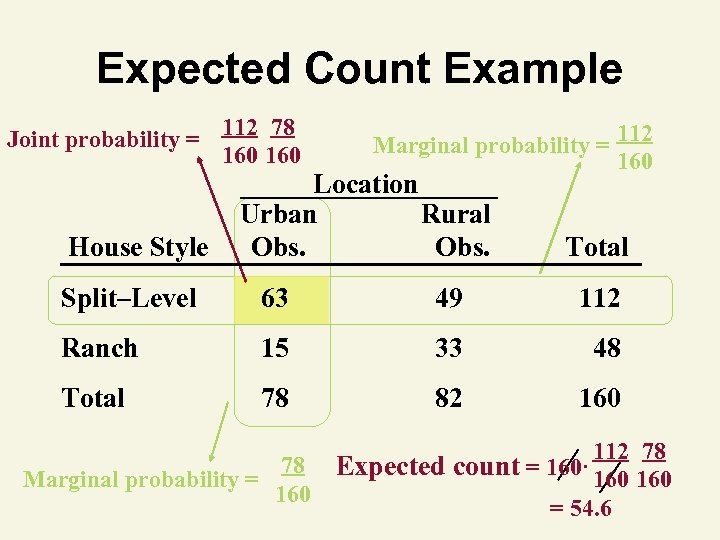Expected Count Example Joint probability = House Style 112 78 160 Marginal probability = 112 160 Location Urban Rural Obs. Total Split–Level 63 49 112 Ranch 15 33 48 Total 78 82 160 Marginal probability = 78 160 112 78 Expected count = 160· 160 = 54. 6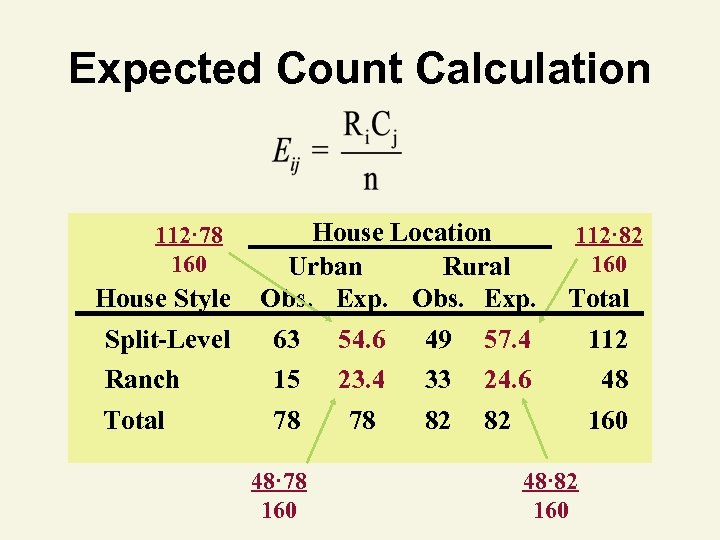Expected Count Calculation 112· 78 160 House Style House Location Urban Rural Obs. Exp. 112· 82 160 Total Split-Level 63 54. 6 49 57. 4 112 Ranch 15 23. 4 33 24. 6 48 Total 78 78 82 82 48· 78 160 48· 82 160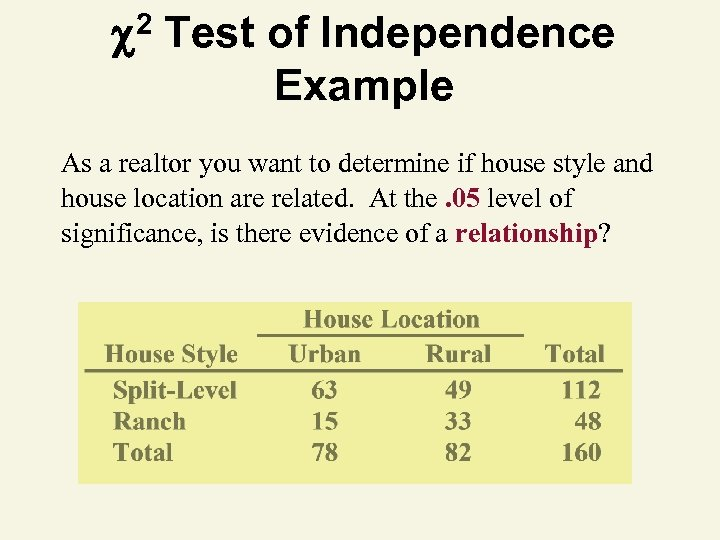2 Test of Independence Example As a realtor you want to determine if house style and house location are related. At the. 05 level of significance, is there evidence of a relationship?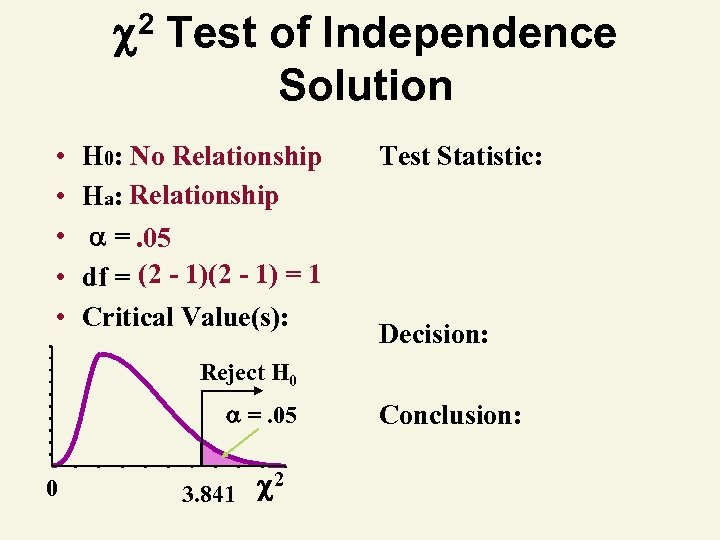2 Test of Independence Solution • • • H 0: No Relationship Ha: Relationship =. 05 df = (2 - 1) = 1 Critical Value(s): Test Statistic: Decision: Reject H 0 =. 05 0 3. 841 2 Conclusion: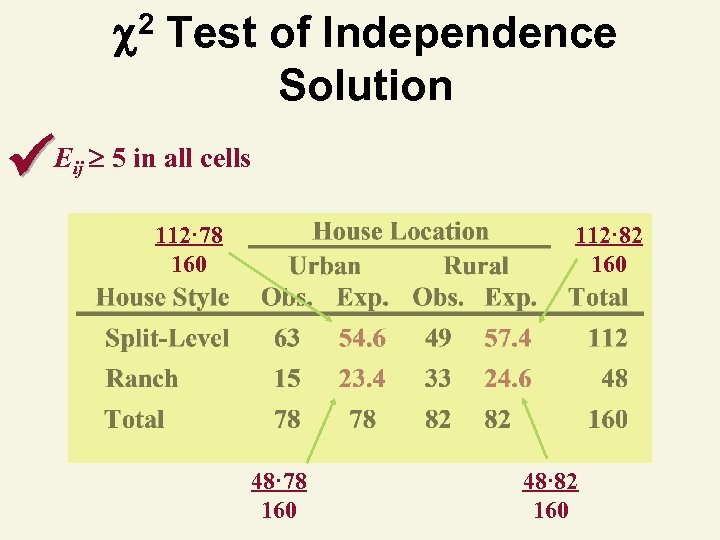2 Test of Independence Solution Eij 5 in all cells 112· 78 160 112· 82 160 48· 78 160 48· 82 160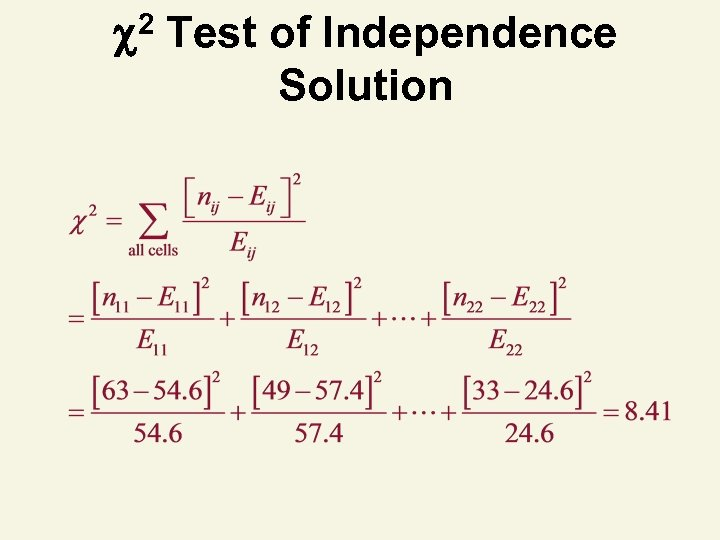2 Test of Independence Solution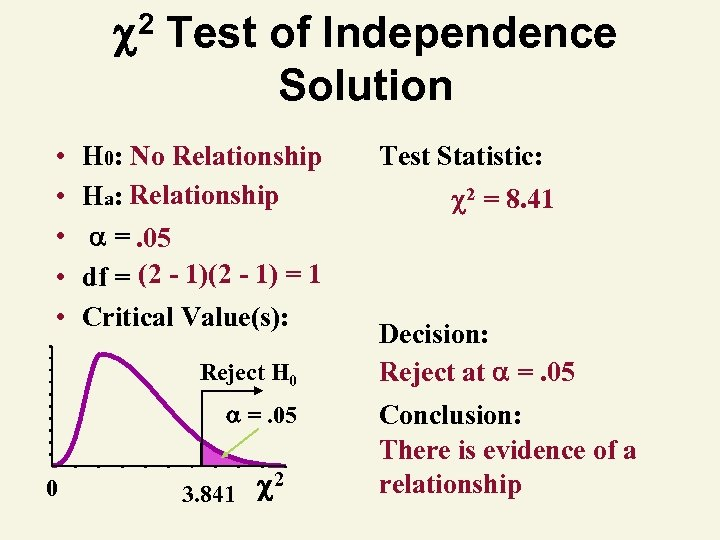2 Test of Independence Solution • • • H 0: No Relationship Ha: Relationship =. 05 df = (2 - 1) = 1 Critical Value(s): Reject H 0 =. 05 0 3. 841 2 Test Statistic: 2 = 8. 41 Decision: Reject at =. 05 Conclusion: There is evidence of a relationship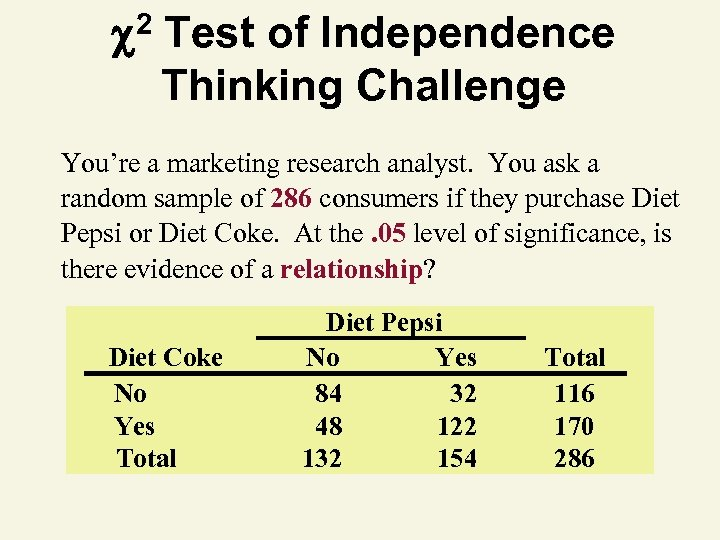2 Test of Independence Thinking Challenge You’re a marketing research analyst. You ask a random sample of 286 consumers if they purchase Diet Pepsi or Diet Coke. At the. 05 level of significance, is there evidence of a relationship? Diet Coke No Yes Total Diet Pepsi No Yes 84 32 48 122 132 154 Total 116 170 286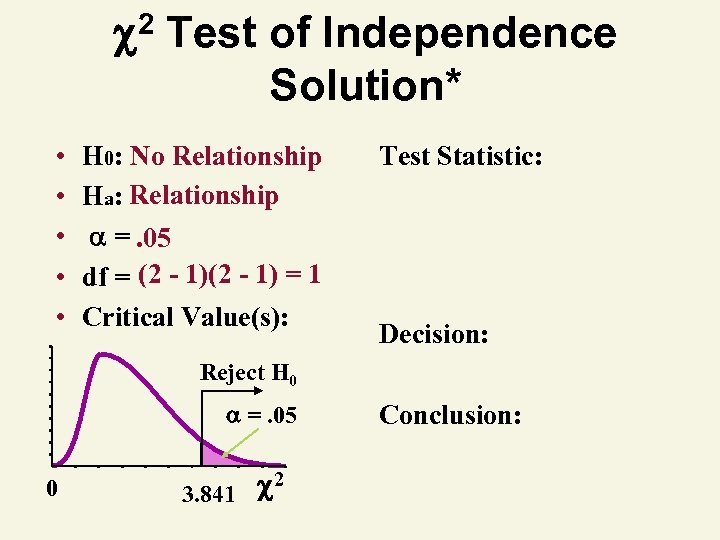2 Test of Independence Solution* • • • H 0: No Relationship Ha: Relationship =. 05 df = (2 - 1) = 1 Critical Value(s): Test Statistic: Decision: Reject H 0 =. 05 0 3. 841 2 Conclusion: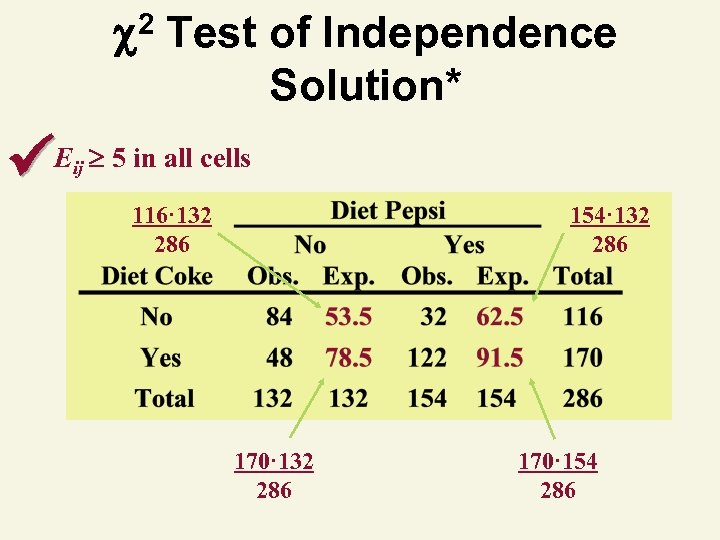2 Test of Independence Solution* Eij 5 in all cells 116· 132 286 154· 132 286 170· 154 286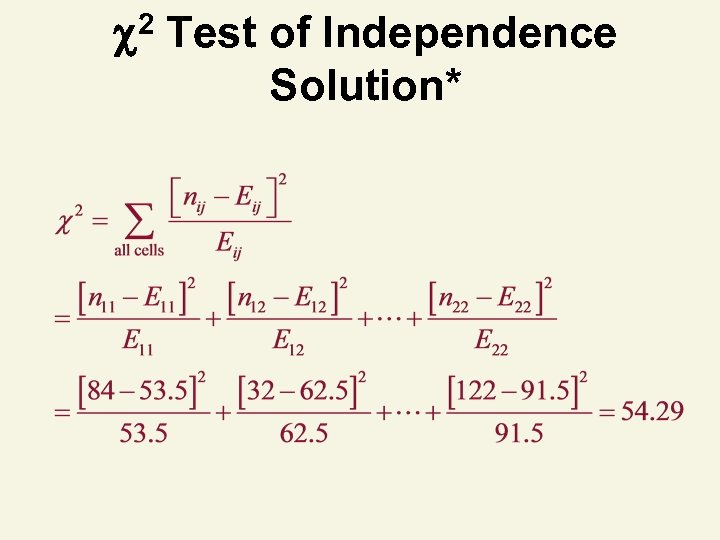2 Test of Independence Solution*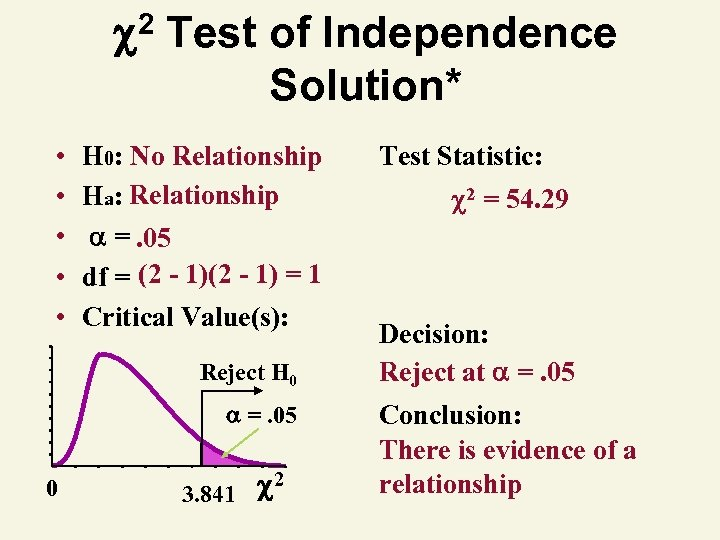2 Test of Independence Solution* • • • H 0: No Relationship Ha: Relationship =. 05 df = (2 - 1) = 1 Critical Value(s): Reject H 0 =. 05 0 3. 841 2 Test Statistic: 2 = 54. 29 Decision: Reject at =. 05 Conclusion: There is evidence of a relationship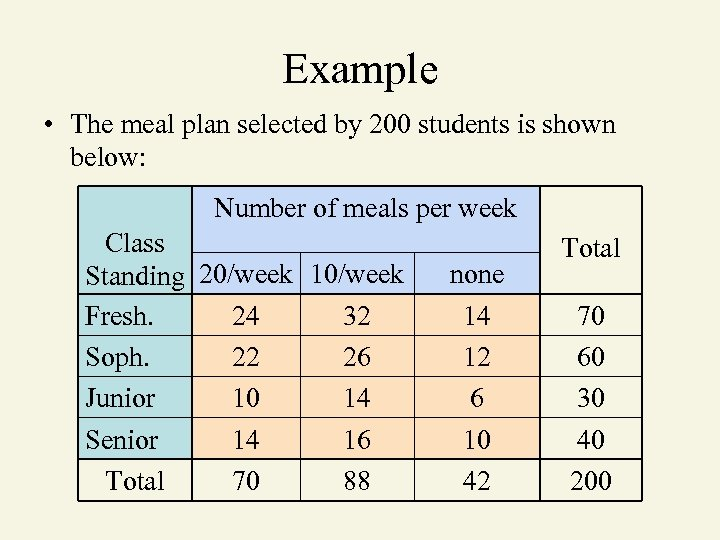Example • The meal plan selected by 200 students is shown below: Number of meals per week Class Standing 20/week 10/week Fresh. 24 32 Soph. 22 26 Junior 10 14 Senior 14 16 Total 70 88 none 14 12 6 10 42 Total 70 60 30 40 200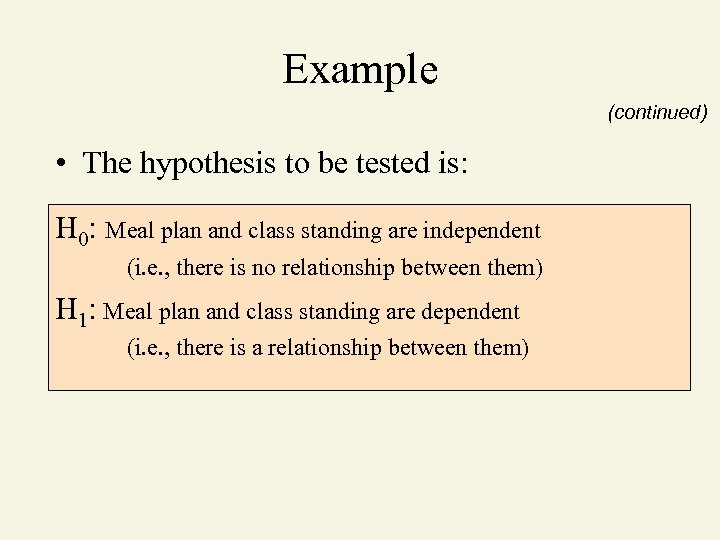Example (continued) • The hypothesis to be tested is: H 0: Meal plan and class standing are independent (i. e. , there is no relationship between them) H 1: Meal plan and class standing are dependent (i. e. , there is a relationship between them)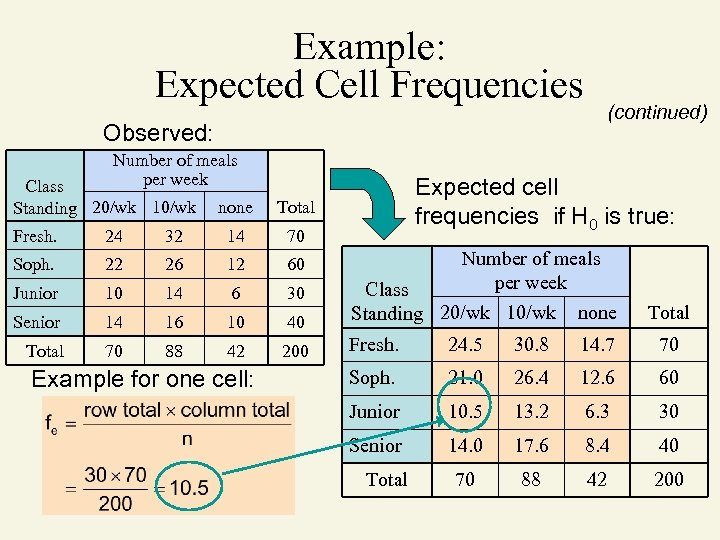Example: Expected Cell Frequencies (continued) Observed: Number of meals per week Class Standing 20/wk 10/wk none Expected cell frequencies if H 0 is true: Total Fresh. 24 32 14 70 Soph. 22 26 12 60 Junior 10 14 6 30 Senior 14 16 10 Total 70 88 42 Example for one cell: Number of meals per week 40 Class Standing 20/wk 10/wk none Total 200 Fresh. 24. 5 30. 8 14. 7 70 Soph. 21. 0 26. 4 12. 6 60 Junior 10. 5 13. 2 6. 3 30 Senior 14. 0 17. 6 8. 4 40 70 88 42 200 Total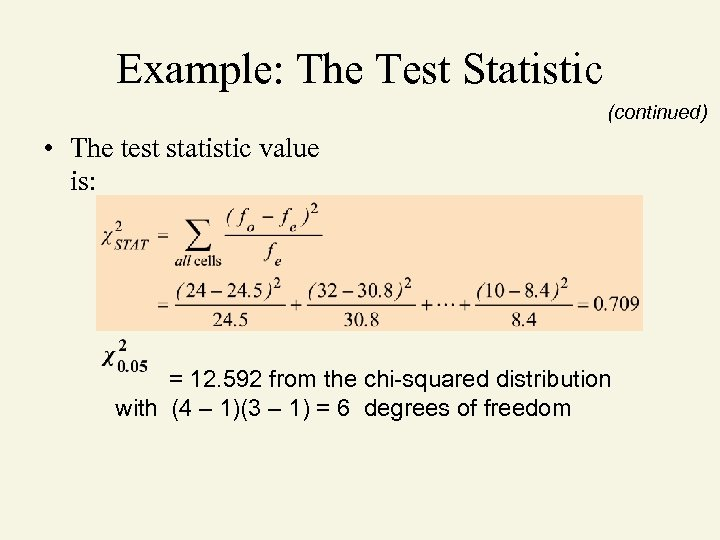Example: The Test Statistic (continued) • The test statistic value is: = 12. 592 from the chi-squared distribution with (4 – 1)(3 – 1) = 6 degrees of freedom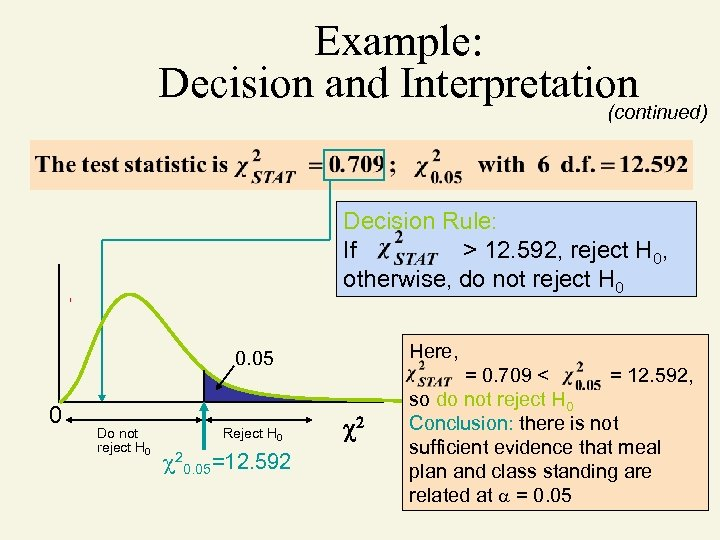Example: Decision and Interpretation (continued) Decision Rule: If > 12. 592, reject H 0, otherwise, do not reject H 0 Here, 0. 05 0 Do not reject H 0 Reject H 0 20. 05=12. 592 2 = 0. 709 < = 12. 592, so do not reject H 0 Conclusion: there is not sufficient evidence that meal plan and class standing are related at = 0. 05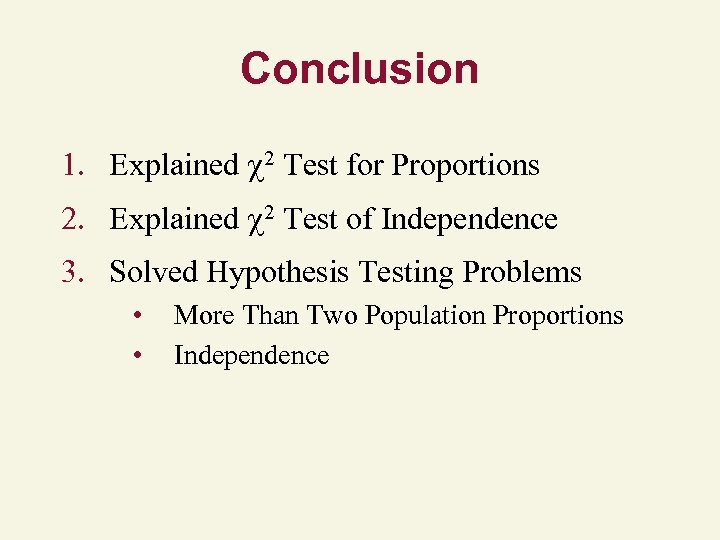Conclusion 1. Explained 2 Test for Proportions 2. Explained 2 Test of Independence 3. Solved Hypothesis Testing Problems • • More Than Two Population Proportions Independence